# Number to Words Converter in Excel - International Format

To convert a Number in Words in MS Excel you have to use Macro, It's very simple to make with few steps, you can easily create it. Just once you have to follow steps given below and you will get a Permanent Formula/Function for Number to word's converter. It can be use as function, so you can convert any column data just by drag and drop the formula.

convert number to words in international format

Steps for Number to Word Converter in MS Excel - International (Million, Billion Format)

Step 1 - Start MS Excel

Step 2 - Just Type Sample Number's in one column (see below image) to convert into Words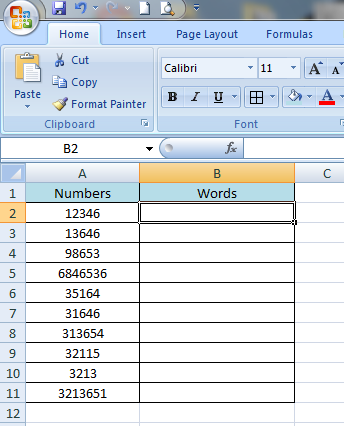Step 3 - Press ALT+F11 to start the Visual Basic Editor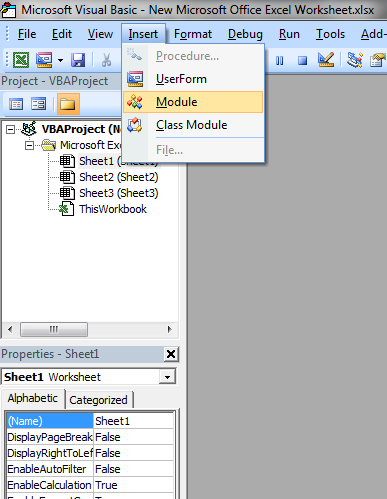Step 4 - You will see a Window is Open, Now copy-paste following code in this window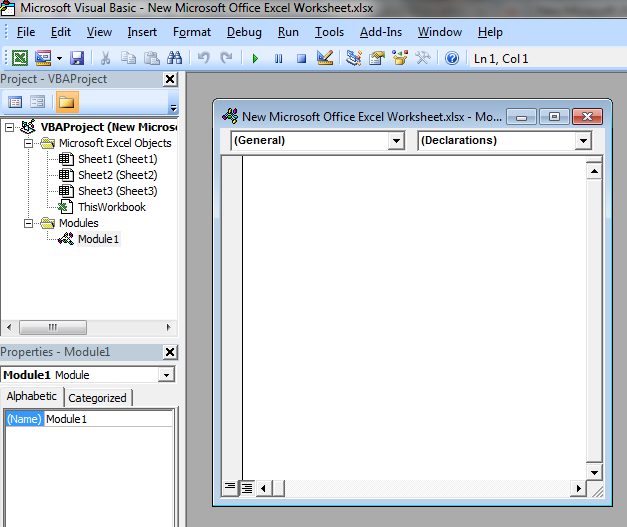``Option Explicit'Main FunctionFunction SpellNumber(ByVal MyNumber)    Dim Dollars, Cents, Temp    Dim DecimalPlace, Count    ReDim Place(9) As String    Place(2) = " Thousand "    Place(3) = " Million "    Place(4) = " Billion "    Place(5) = " Trillion "    ' String representation of amount.    MyNumber = Trim(Str(MyNumber))    ' Position of decimal place 0 if none.    DecimalPlace = InStr(MyNumber, ".")    ' Convert cents and set MyNumber to dollar amount.    If DecimalPlace > 0 Then        Cents = GetTens(Left(Mid(MyNumber, DecimalPlace + 1) & _                  "00", 2))        MyNumber = Trim(Left(MyNumber, DecimalPlace - 1))    End If    Count = 1    Do While MyNumber <> ""        Temp = GetHundreds(Right(MyNumber, 3))        If Temp <> "" Then Dollars = Temp & Place(Count) & Dollars        If Len(MyNumber) > 3 Then            MyNumber = Left(MyNumber, Len(MyNumber) - 3)        Else            MyNumber = ""        End If        Count = Count + 1    Loop    Select Case Dollars        Case ""            Dollars = "No Dollars"        Case "One"            Dollars = "One Dollar"         Case Else            Dollars = Dollars & " Dollars"    End Select    Select Case Cents        Case ""            Cents = " and No Cents"        Case "One"            Cents = " and One Cent"              Case Else            Cents = " and " & Cents & " Cents"    End Select    SpellNumber = Dollars & CentsEnd Function      ' Converts a number from 100-999 into text Function GetHundreds(ByVal MyNumber)    Dim Result As String    If Val(MyNumber) = 0 Then Exit Function    MyNumber = Right("000" & MyNumber, 3)    ' Convert the hundreds place.    If Mid(MyNumber, 1, 1) <> "0" Then        Result = GetDigit(Mid(MyNumber, 1, 1)) & " Hundred "    End If    ' Convert the tens and ones place.    If Mid(MyNumber, 2, 1) <> "0" Then        Result = Result & GetTens(Mid(MyNumber, 2))    Else        Result = Result & GetDigit(Mid(MyNumber, 3))    End If    GetHundreds = ResultEnd Function      ' Converts a number from 10 to 99 into text. Function GetTens(TensText)    Dim Result As String    Result = ""           ' Null out the temporary function value.    If Val(Left(TensText, 1)) = 1 Then   ' If value between 10-19...        Select Case Val(TensText)            Case 10: Result = "Ten"            Case 11: Result = "Eleven"            Case 12: Result = "Twelve"            Case 13: Result = "Thirteen"            Case 14: Result = "Fourteen"            Case 15: Result = "Fifteen"            Case 16: Result = "Sixteen"            Case 17: Result = "Seventeen"            Case 18: Result = "Eighteen"            Case 19: Result = "Nineteen"            Case Else        End Select    Else                                 ' If value between 20-99...        Select Case Val(Left(TensText, 1))            Case 2: Result = "Twenty "            Case 3: Result = "Thirty "            Case 4: Result = "Forty "            Case 5: Result = "Fifty "            Case 6: Result = "Sixty "            Case 7: Result = "Seventy "            Case 8: Result = "Eighty "            Case 9: Result = "Ninety "            Case Else        End Select        Result = Result & GetDigit _            (Right(TensText, 1))  ' Retrieve ones place.    End If    GetTens = ResultEnd Function     ' Converts a number from 1 to 9 into text. Function GetDigit(Digit)    Select Case Val(Digit)        Case 1: GetDigit = "One"        Case 2: GetDigit = "Two"        Case 3: GetDigit = "Three"        Case 4: GetDigit = "Four"        Case 5: GetDigit = "Five"        Case 6: GetDigit = "Six"        Case 7: GetDigit = "Seven"        Case 8: GetDigit = "Eight"        Case 9: GetDigit = "Nine"        Case Else: GetDigit = ""    End SelectEnd Function``

Step 5 - Save this by clicking on save in window with following format, Excel macro Enabled Workbook.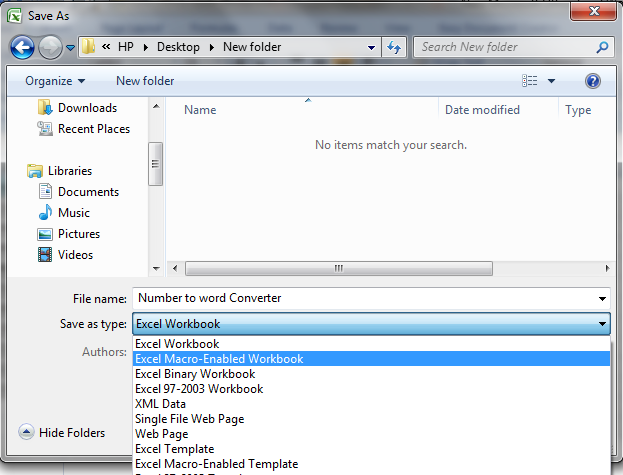Step 6 - Now come again on your original excel sheet on which you have to convert Number in Words.
Step 7 - Just Click on formulas then -> Insert Function Option, a window will open.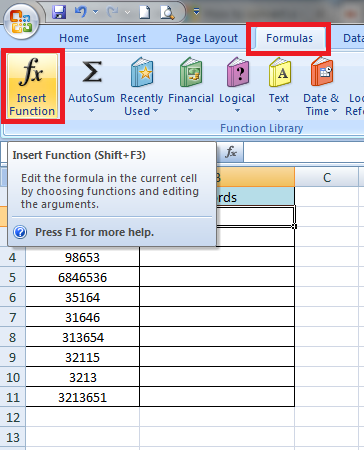Step 8 - In Drop Down Box Select "User Defined".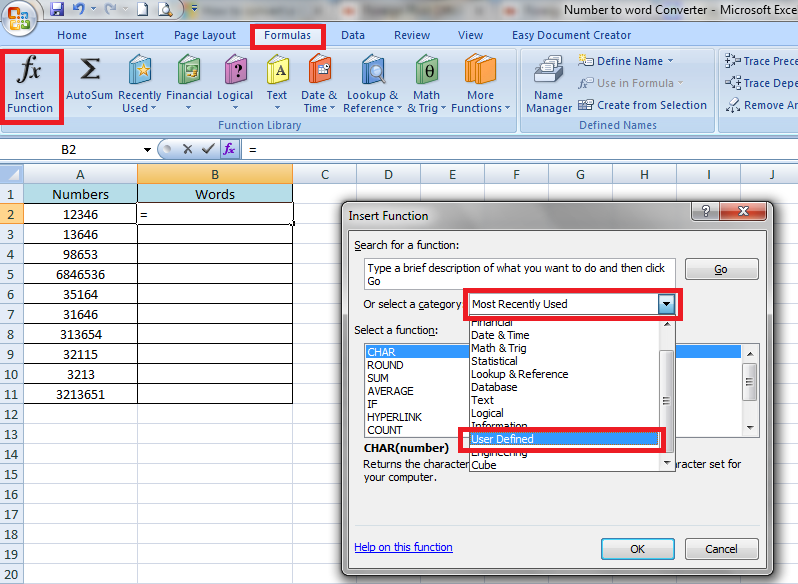Step 9 - Now Select "SpellNumber" and Click on OK.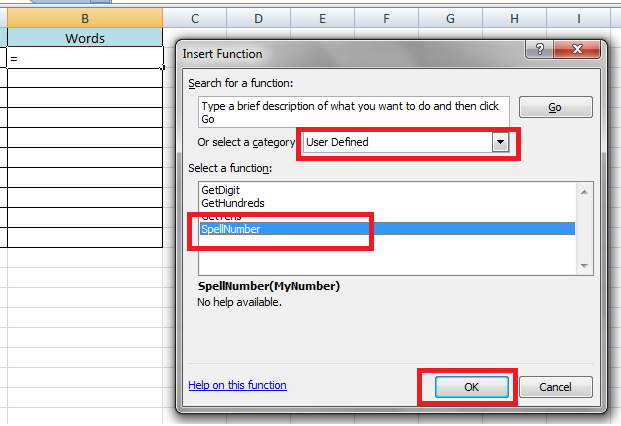Step 10 - Now a New Window will Show Click on button shown as below and Select Number Column A1. Click on OK. You will see Number is converted in Words.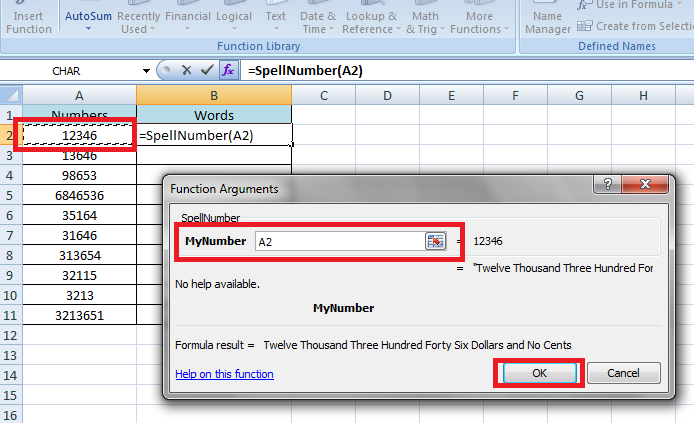Step 11 - Now your number will converted in words. You can drag and drop to apply this converter formula on all of the numbers.Step 12 - Now you can use this Macro Excel sheet to use Formula you have Made.